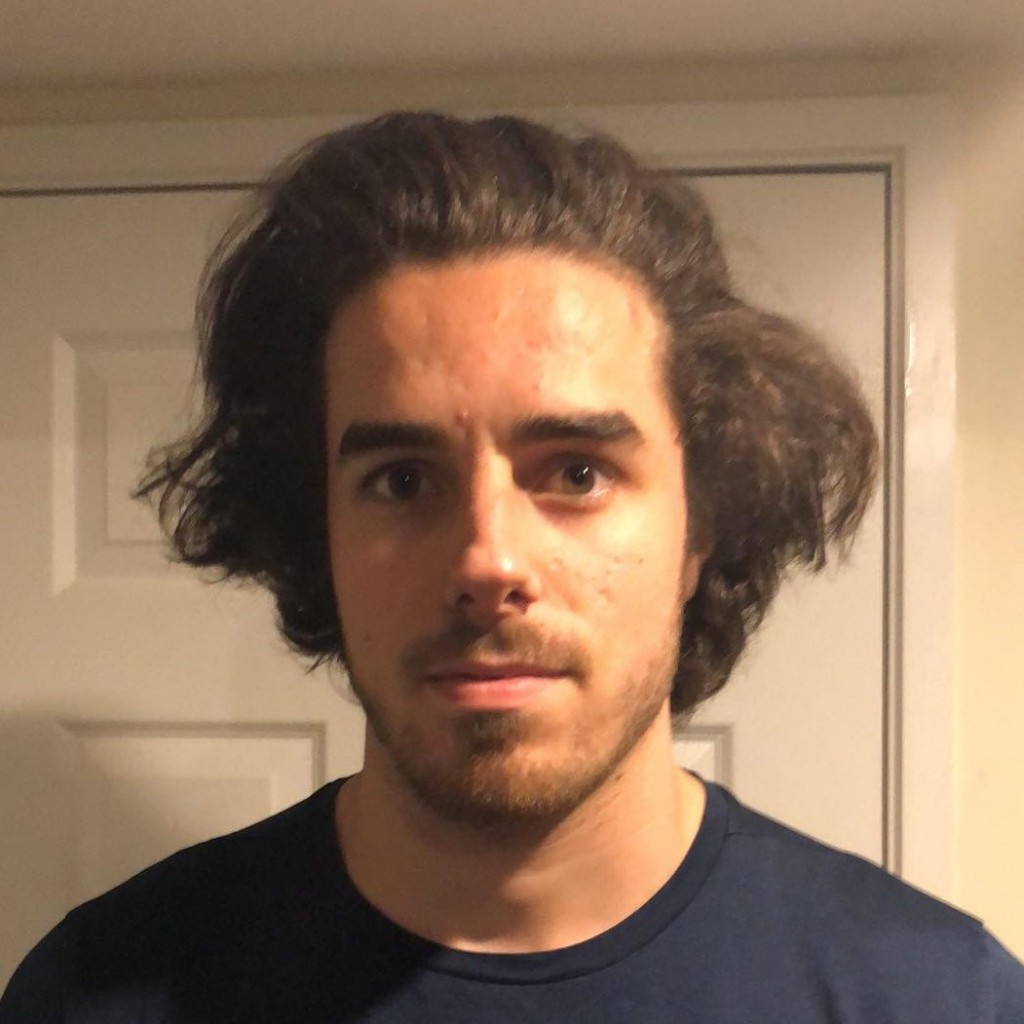I have always been interested in mathematics, as a young primary school student mathematics was simple arithmetic, that is, addition, subtraction, multiplication and division of whole numbers. I specifically remember applying myself to memorising all the multiplication tables from 1 to 12. Mathematics quickly became my favourite subject at school. Going further into early high school and learning about arithmetic with the rational numbers (i.e. adding, subtracting and multiplying fractions) and negative numbers was something I really enjoyed. In this period, I heard the word ‘algebra’, which was always accompanied by a negative tone or groan, from other peers who were in grades above me. I was really curious to study this new field as I had heard it involves the use of ‘letters’ which I had soon learned that they were actually variables. I enjoyed algebra particularly solving simple linear equations. I realised it was important to be proficient in algebra since it was required to solve problems in simple trigonometry and geometry. As I went through the higher grades of high school, I chose to do that highest maths subject (Maths B and C), since maths was my favourite subject I didn’t mind, at all, doing two subjects in Maths. I was completely blown away when our teacher introduced complex numbers as I had never imagined taking the square root of a negative number, at this point I was completely hooked into mathematics. I thought calculus was the coolest thing ever, especially the idea behind derivatives and integrals. We were also introduced to basic concepts in linear algebra, group theory and number theory which I found kind of quirky, especially number theory and group theory which involved mathematical proofs. When I had finished year 12, I naively thought I had learned most of the mathematics there is to know… I soon realised I hadn’t even started to scratch the surface.

It was obvious to me that I only liked two subjects, Maths and Science, so I decided to do a double degree in Maths (applied and computational mathematics) and Science (physics) at QUT. The next four years I had learned a lot about Maths, but at the same time, I learned how much I didn’t know about Mathematics. In my final semester I realised, as I was taking a course in Dynamical systems, which I really enjoyed, that I was much more interested in pure mathematics than computational mathematics. So, I asked the lecturer of this course if I could do a 10-week research project (AMSI scheme) related to dynamical systems under his supervision to which he kindly accepted. The experience over the 10 weeks was an excellent one and I am grateful for it has given me the opportunity to peak through the door of pure mathematics. This experience inspired me to take a bit of a turn in my academic career, and that is to take some pure mathematics courses with the hope of eventually doing post-graduate studies in pure mathematics.

Stefano Giannini
Queensland University of TechnologyView Stefano Giannini
Profile